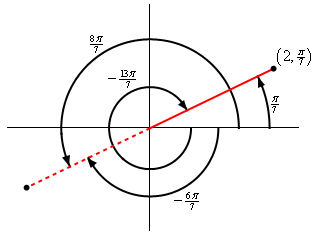Paul's Online Notes
Home / Calculus II / Parametric Equations and Polar Coordinates / Polar Coordinates
Show Mobile Notice Show All Notes Hide All Notes
Mobile Notice
You appear to be on a device with a "narrow" screen width (i.e. you are probably on a mobile phone). Due to the nature of the mathematics on this site it is best views in landscape mode. If your device is not in landscape mode many of the equations will run off the side of your device (should be able to scroll to see them) and some of the menu items will be cut off due to the narrow screen width.

### Section 9.6 : Polar Coordinates

1. For the point with polar coordinates $$\displaystyle \left( {2,\frac{\pi }{7}} \right)$$ determine three different sets of coordinates for the same point all of which have angles different from $$\displaystyle \frac{\pi }{7}$$ and are in the range $$- 2\pi \le \theta \le 2\pi$$.

Show All Steps Hide All Steps

Start Solution

This problem is really as exercise in how well we understand the unit circle. Here is a quick sketch of the point and some angles.We can see that the negative angle ending at the solid red line that is in the range specified in the problem statement is simply $$\frac{\pi }{7} - 2\pi = - \frac{{13\pi }}{7}$$.

If we extend the solid line into the third quadrant (i.e. the dashed red line) then the positive angle ending at the dashed red line is $$\frac{\pi }{7} + \pi = \frac{{8\pi }}{7}$$. Likewise, the negative angle ending at the dashed red line is $$\frac{\pi }{7} - \pi = - \frac{{6\pi }}{7}$$.

With these angles getting the other three points should be pretty simple.

Show Step 2

For the first “new” point we can use the negative angle that ends on the solid red line to get the point.

$\require{bbox} \bbox[2pt,border:1px solid black]{{\left( {2, - \frac{{13\pi }}{7}} \right)}}$ Show Step 3

For the remaining points recall that if we use a negative $$r$$ then we go “backwards” from where the angle ends to get the point. So, if we use $$r = - 2$$, any angle that ends on the dashed red line will go “backwards” into the first quadrant 2 units to get to the point.

This gives the remaining two points using both the positive and negative angle ending on the dashed red line,

$\require{bbox} \bbox[2pt,border:1px solid black]{{\left( { - 2, - \frac{{6\pi }}{7}} \right)}}\hspace{0.25in}\hspace{0.25in}\hspace{0.25in}\require{bbox} \bbox[2pt,border:1px solid black]{{\left( { - 2,\frac{{8\pi }}{7}} \right)}}$